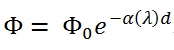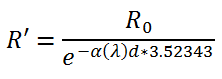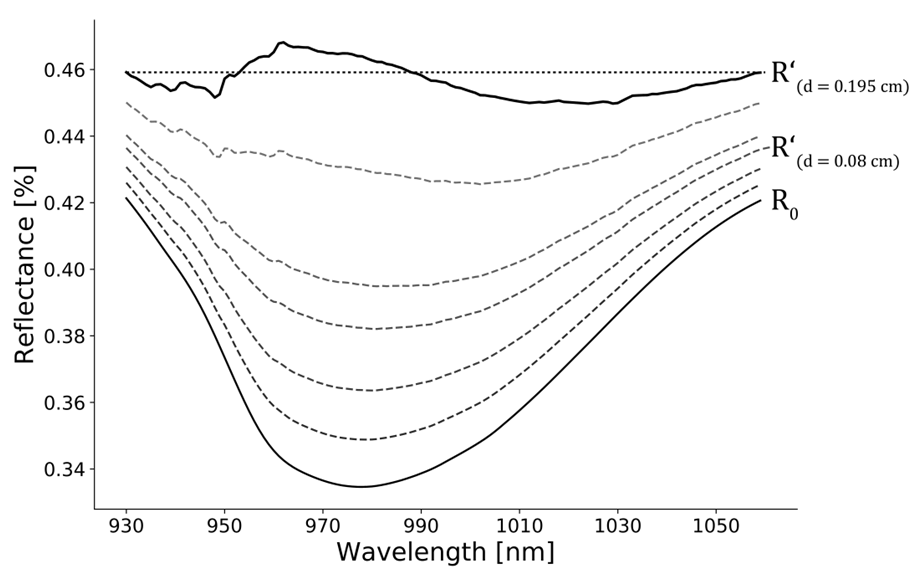# Plant Water Retrieval (PWR)¶

The Plant Water Retrieval (PWR) tool uses is an efficient algorithm to directly extract quantitative water content information in [g cm-2] or [cm] from hyperspectral images. It applies the Beer-Lambert law  to inversely determine the optical thickness d of the water layer responsible for the water absorption feature at 970 nm using water absorption coefficients $${\alpha}$$ for pure liquid water. $${\Phi}$$ 0 denotes the incident radiation intensity and $${\Phi}$$ is the attenuated radiation intensity.After calibration over various validation datasets (refer to Wocher et al. (2018) for detailed information) the final PWR equation  is as follows:with R 0 being the measured reflectance. The algorithm stops when the sum of the residuals to the assumed dry reflectance (Figure 1) is reached.Figure 1: Determination of optically active water thickness d from a measured spectrum through minimization of residuals to assumed dry reflectance line (dotted line).

## Getting Started¶

1. Select a GDAL readable hyperspectral input image via the button and define a location for the output image .
2. If you need to speed up the calculation a NDVI threshold for vegetation pixels can be assigned.
3. Select an Output No Data value for the output image.
4. If your input image is of integer type, assign a division factor to obtain relative reflectances expected by the algorithm.
5. Click Run to start the plant water retrieval algorithm.